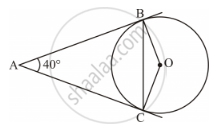Share

# In Figure 2, Ab and Ac Are Tangents to the Circle with Centre O Such that ∠Bac = 40°. Then ∠Boc is Equal to - Mathematics

Course
ConceptTangent to a Circle

#### Question

In Figure 2, AB and AC are tangents to the circle with centre O such that

∠BAC = 40°. Then ∠BOC is equal to• A. 40°

• B. 50°

• C. 140°

• D. 150°

#### Solution

It is known that the tangent is perpendicular to the radius through the point of contact.

∴ ∠ABO = ∠ACO = 90°

Using angle sum property in quadrilateral ABOC:

∠ABO + ∠BOC + ∠ACO + ∠BAC = 360°

⇒ 90° + ∠BOC + 90° + 40° = 360°

⇒ ∠BOC + 220° = 360°

⇒ ∠BOC = 360° − 220° = 140°